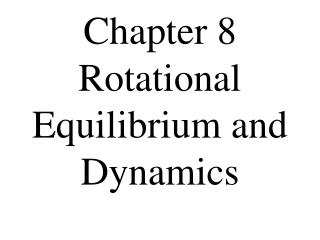DownloadDownload PresentationChapter 8 Rotational Equilibrium and Dynamics

# Chapter 8 Rotational Equilibrium and Dynamics

Download Presentation## Chapter 8 Rotational Equilibrium and Dynamics

- - - - - - - - - - - - - - - - - - - - - - - - - - - E N D - - - - - - - - - - - - - - - - - - - - - - - - - - -
##### Presentation Transcript

1. Chapter 8 Rotational Equilibrium and Dynamics

2. 8-1 Torque

3. Torque(t) A quantity that measures the ability of a force to rotate an object around some axis. t  Greek letter ‘tau’

4. Net Torque produces rotation. Just like a net force causes motion/acceleration.

5. Torque depends upon a force and the length of the lever arm.

6. How easily an object rotates depends not only on ‘how much’ force is applied, but also where the force is applied.

7. Lever Arm The perpendicular distance from the axis of rotation to a line drawn along the direction of the force.

8. l

9. t = Fd(sinq) Metric Units of torque are Newton-meters (N-m)

10. Torque, like displacement and force, is a vector quantity. Since torque is a rotational motion it has two directions:

11. Counterclockwise (CCW), which is positive. • Clockwise (CW), which is negative.

12. Ex 1: Sean has a flat and is changing his tire, to remove the lug nuts he applies a force of 25 N on the tire iron at an angle of 87o. What is the torque produced if the tire iron is 0.6 m long?

13. G: d = 0.6 m, F= 25 N, q = 87o U: t = ? E: t = Fd(sinq) S: t = (25 N)(0.6 m)(sin 87o) S: t =14.98 N-m

14. Ex 2: What angle produces a torque of 400 N-m, if the force applied is 505 N at a distance from the axis of rotation of 0.82 m?

15. G: t = 400 N-m, F = 505 N, d =0.82 m U: q = ? E:

16. G: t = 400 N-m, F = 505 N, d =0.82 m U: q = ? E:

17. 8-2 Rotation and Inertia

18. Point mass Where all the mass is assumed to be located in one point.

19. Center of Mass The point at which all the mass of a body can be considered to be concentrated when analyzing translational motion.

20. Rotational and translational motion can be combined. We use the the center of mass, as a reference, to analyze its translation motion.

21. Center of Gravity The position at which the gravitational force acts on an extended object as if it were a point mass.

22. Toppling • If the Center of Gravity (CG) is above the support area, then the object will remain upright. • If the CG extends outside the support area, the object will topple.

23. Unstable Equilibrium Is when any movement/ displacement of a balanced object lowers the CG.

24. Stable Equilibrium • Is when any motion/displacement of a balanced object raises its CG.

25. Neutral Equilibrium • Is when any motion/displacement of a balanced object neither raises nor lowers its CG.

26. Moment of Inertia (MOI) The tendency of a body to rotate freely about a fixed axis to resist a change in rotational motion.

27. MOI is the rotational analog of mass. Very similar to mass, but MOI is not an intrinsic property of an object.

28. It depends upon the object’s mass and the distribution of mass around the axis of rotation.

29. The farther the mass is, on average from the axis of rotation, the greater the the object’s MOI and the more difficult it is to rotate the object.

30. Calculating the MOI Pg 285: Table 8-1 has equations/formulas for a few common shapes. M – mass in kilograms R – radius in meters l – length in meters

31. Units for MOI are kg-m2

32. For an object to be in complete equilibrium, requires zero net force and zero net torque.

33. If the net force is zero the object is in translational equilibrium. (1st Condition of Equilibrium)

34. If the net torque is zero, than it is in rotational equilibrium. (2nd Condition for Equilibrium)

35. 8-3 Rotational Dynamics

36. Newton’s 2nd Law for Rotation F = ma We know in a rotational system, torque is a function of force, also:

37. 1. MOI replaces the mass. 2. Acceleration is replaced with angular acceleration.

38. Net torque = MOI x Angular acel.

39. Remember that if the net torque is zero, that the object can still be rotating, just at a constant velocity.

40. Ex 4: Simon decides to ride the ‘Gravitron’, it has a radius of 3 m, if his mass is 79.4 kg. What is the net torque produced when his angular acceleration is 7 rads/sec2?

41. G: R = 3 m, M= 79.4 kg, a = 7 rads/sec2 U: tnet = ? E: tnet = Ia We are going to assume he is a point mass.

42. MOI, I = MR2 S:tnet = MR2a S: tnet = (79.4)(3)2(7) S: tnet = 5002.2 N-m

43. Ex 5: In, 1995, a fully functional pencil with a mass of 24 kg and a length of 2.74 m was made. Suppose this pencil is suspended at its midpoint and a force of 1.8 N is applied perpendicular to its end, causing it to rotate. What is the angular acceleration of the pencil?